# Sequences

Here you will learn about sequences, including what they are, examples of sequences, and how to find and extend the pattern rule.

Students will first learn about sequences as part of operations and algebraic thinking in 4th and 5th grade. They continue to build on this knowledge in middle school and high school.

## What are sequences?

Sequences (numerical patterns) are sets of numbers that follow a particular pattern or rule to get from number to number. Each number is called a term in a pattern. Two types of sequences are arithmetic and geometric.

An arithmetic sequence is a number pattern where the rule is addition or subtraction. To create the rule, look for the common difference between the terms and then decide if the terms are going up or down.

The rule should be general enough that it helps you find the next terms of the sequence.

For example,

Rule: Subtract 1 each time.

For example,

You can also look for patterns within the terms. In this pattern, the terms alternate between odd and even. Since 3 is being added each time the terms alternate between being odd and even.

For example,

Here, you can see the ones digit of each term alternates between being a 4 and a 9 .

Within two or more arithmetic sequences, there are also patterns. Let’s look at the two addition patterns side by side.

For example,

The difference between the terms starts at 0 and then goes up by 2 each time. Why is this? The first difference is 0, because both patterns start at 4.

Since one pattern +3 and one pattern +5, the +5 pattern will always add 2 more than the +3 pattern. This causes the difference to grow by 2 each time.

A geometric sequence is a number pattern where the rule is multiplication or division.

For example,

Rule: Multiply the previous term by 2 .

For example,

Rule: Divide the previous term by 2 .

*Note, this page will highlight rules that involve whole numbers only. However, for geometric rules, there is always an additional rule involving fractions that is correct.

For example, the rule above could also be ‘Multiply the previous term by \, \cfrac{1}{2} .’

Two special mathematical sequences are triangular numbers and the fibonacci sequence.

Triangular numbers are numbers that can visually form a triangular dot pattern:

Rule: Add a bottom row with 1 more circle each time.

When looking at just the numbers, triangular numbers start at 1 and have one more added each time:

Rule: Start at 1 and add 2, then add one more each time.

The first term in the fibonacci sequence is 0 and the second term is 1. Then the pattern is created, starting with the third term by adding the previous two terms together to find the next term.

The numbers in the fibonacci sequence are also referred to as fibonacci numbers. The fibonacci sequence is used to create the golden ratio, which forms a spiral and is seen throughout nature.

### What are sequences?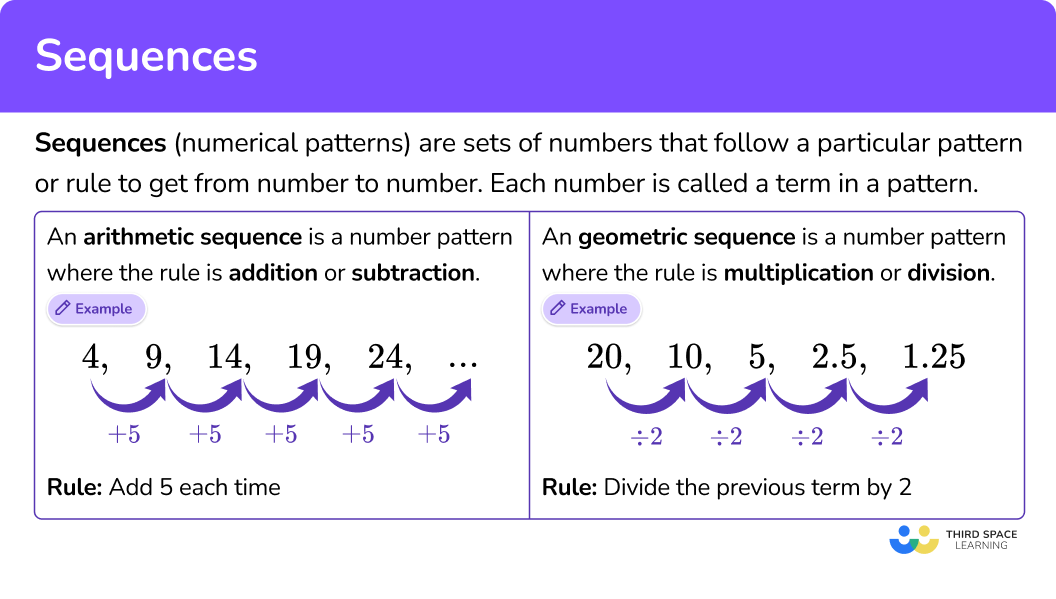## Common Core State Standards

How does this relate to 4th grade math and 5th grade math?

• Grade 4 – Operations and Algebraic Thinking (4.OA.C.5)
Generate a number or shape pattern that follows a given rule. Identify apparent features of the pattern that were not explicit in the rule itself.

For example, given the rule “Add 3 ” and the starting number 1, generate terms in the resulting sequence and observe that the terms appear to alternate between odd and even numbers. Explain informally why the numbers will continue to alternate in this way.

• Grade 5 – Operations and Algebraic Thinking (5.OA.B.3)
Generate two numerical patterns using two given rules. Identify apparent relationships between corresponding terms. Form ordered pairs consisting of corresponding terms from the two patterns, and graph the ordered pairs on a coordinate plane.

For example, given the rule “Add 3 ” and the starting number 0, and given the rule “Add 6 ” and the starting number 0, generate terms in the resulting sequences, and observe that the terms in one sequence are twice the corresponding terms in the other sequence. Explain informally why this is so.

## How to use sequences

In order to identify and extend sequences:

1. Identify the rule.
2. Use the rule to extend the sequence.
3. State and explain any patterns within the terms.

In order to compare sequences:

1. Identify the rule of each sequence.
2. Find a pattern between the related terms of each sequence.
3. Use the pattern to write a comparison statement.

## Sequences examples

### Example 1: arithmetic sequence

What are the next three terms in the pattern?

5, 9, 13, 17, 21…

1. Identify the rule.

2Use the rule to extend the sequence.

Add 4 each time to extend the pattern to the next three terms.

3State and explain any patterns within the terms.

The terms are all odd numbers. The terms alternate ending in 5, 9, 3, 7, and 1.

Since 4 (even number) is being added each time to the last term (odd number), the terms will stay odd even + odd = odd.

### Example 2: geometric sequence

What are the next three terms in the pattern?

3, 6, 12, 24, 48…

Rule: Multiply the previous term by 2 .

Multiply the last term by 2 to extend the pattern to the next three terms.

Except for the first term, all the terms are even. The first term is odd, but multiplying it by 2 (even), makes the next term even.

From then on, it is always an even term times 2 → even \times even = even.

### Example 3: geometric sequence

What are the next three terms in the pattern?

1, 4, 16, 64, 256, …

Rule: Multiply the previous term by 4 .

Multiply the last term by 4 to extend the pattern to the next three terms.

Except for the first term, all the terms are even. The first term is odd, but multiplying it by 4 (even), makes the next term even.

From then on, it is always an even term times 4 → even \times even = even.

### Example 4: arithmetic sequence

What are the next three terms in the pattern?

54, 49, 44, 39, 34…

Rule: Subtract 5 each time.

Subtract 5 each time to extend the pattern to the next three terms.

The terms alternate ending in 4 and 9. Since subtracting 5 twice is like subtracting 10, every other term decreases by ten – leaving the ones position the same.

The terms also alternate between odd and even. When subtracting 5 (odd number) from an even number, the result is odd even odd = odd. When subtracting 5 from an odd number, the result is even odd odd = even.

### Example 5: compare arithmetic sequences

Compare the following sequences:

0, 2, 4, 6, 8… \, and \, 0, 12, 24, 36, 48…

Look at the related terms for each sequence. What do you notice?

Both sequences start at 0. If you multiply the left column by 6, you get the terms in the right column.

The terms in the second sequence are 6 times the terms in the first sequence.

This is because using the rule +12 is six times more than using the rule +2.

### Example 6: compare arithmetic sequences

Compare the following sequences:

78, 71, 64, 57, 50… \, and \, 78, 76, 74, 72, 70…

Look at the related terms for each sequence. One way to find a pattern is to look at the difference between each term.

The first difference is 0, because both patterns start at 78. Since one pattern -7 and one pattern -2, the -7 pattern will always subtract 5 more than the -2 pattern.

This is why the difference grows by 5 each time.

### Teaching tips for sequences

• Introduce arithmetic and geometric sequences together. Identifying the rule and extending a sequence requires students to notice how the pattern is changing from term to term. It is important to get students thinking from the get-go whether or not a sequence is arithmetic or geometric. Showing them only arithmetic sequences first can lead to misconceptions and does not challenge them to think multiplicatively.

• Allow students to create their own patterns and have classmates look for the rule and extend them. This keeps students engaged while deepening their understanding by challenging them to create.

• Have students explore math patterns in nature so that they can make connections between the math concepts and the world around them.

### Easy mistakes to make

• Confusing the kinds of sequences
At an elementary level, students work recursively with sequences. While it is not necessary to teach this term, it is important to draw students’ attention to how the rule is being defined – term to term. This can be confusing when an arithmetic sequence is a list of multiples.

For example,
What is the rule for the pattern 4, 8, 12, 16, 20?
To find the next term in the sequence, you need to add 4, not multiply.
While the terms are multiples of 4, in order for this to be the rule, you would need to have corresponding inputs and outputs, such as…

• Identifying the wrong rule
It is important to look at all terms in a sequence before defining the rule.
Looking at just two can lead to mistakes when creating the rule.
For example,
What is the rule for the pattern 4, 8, 12, 16, 20?

• Making a calculation error
Since working with patterns requires operating with numbers, there is always room for errors. It is important to double-check all work and consider if your answers are reasonable.

### Sequences practice questions

1. What are the next three terms in the pattern?

256, 128, 64, 32, 16…

32, 64, 12810, 5, 212, 8, 48, 4, 2Identify the rule.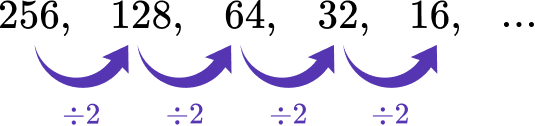Rule: Divide the previous term by 2 .

Divide the last term by 2 to extend the pattern to the next three terms.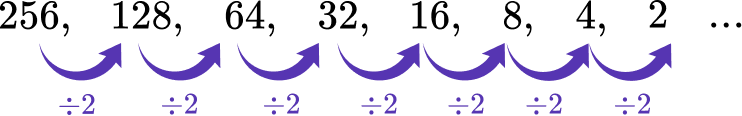2. What is the rule for the pattern?

42, 35, 28, 21, 14, 7…

Subtract 7 each timeDivide by 7 each timeSubtract 6 each timeDivide by 6 each timeIdentify the rule.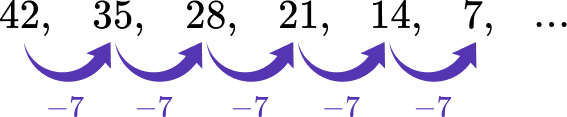Rule: Subtract 7 each time.

3. What is the first term?

?, 15, 24, 33, 42, 51…

46911Identify the rule.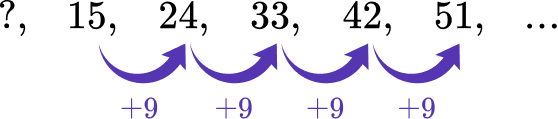Rule: Add 9 to the previous term.

Think about the number where adding 9 will equal the term 15. You can also use the rule backwards, by subtracting 9 to find the term before 15.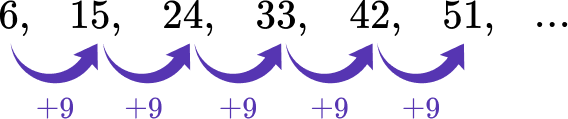4. Starting number: 134

Which statement is true about the terms of the sequence described above?

All the terms are evenAll the terms are oddThe terms alternate between even and oddThe first term is even and the rest are oddExtend the sequence based on the starting number and the rule.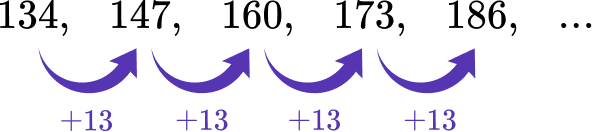Since 13 (odd number) is being added each time, and odd + even = odd and odd + odd = even, the terms alternate between even and odd.

5. Which statement correctly compares the terms of the sequences?

Sequence A: 0, 6, 12, 18, 24, 30…

Sequence B: 0, 12, 24, 36, 48, 60…

The terms start odd, then become even.The terms are being multiplied by 6 and 12.The terms for the rule +12 are 6 more than for the rule +6.The terms for the rule +12 are double the terms for the rule +6.Identify the rule for Sequence A.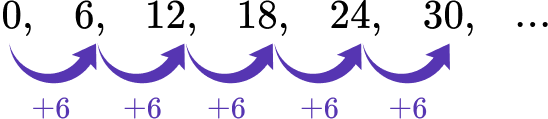Rule: Add 6 from the previous term.

Identify the rule for Sequence B.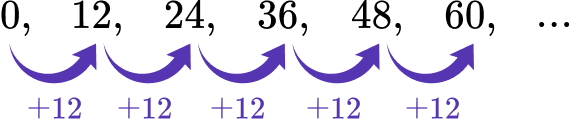Rule: Add 12 from the previous term.

Look at the related terms for each sequence. What do you notice?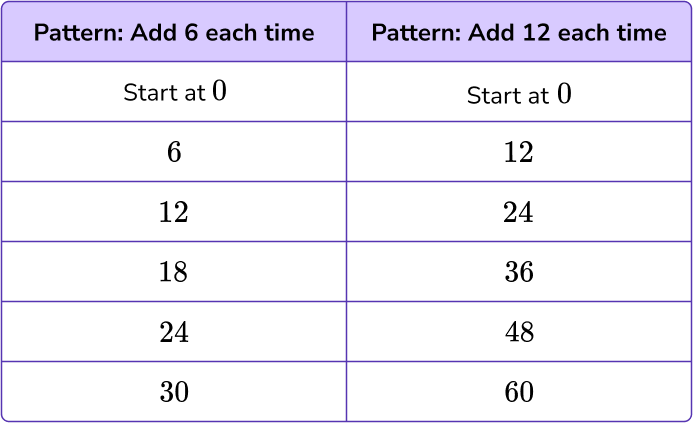Both sequences start at 0. If you multiply the left column by 2, you get the terms in the right column.

The rule +12 is double the rule +6.

6. Which statement correctly compares the difference between the terms of the sequences?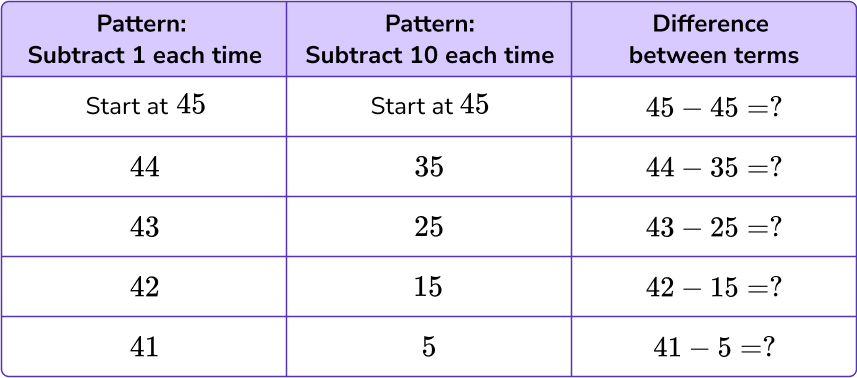Since both patterns start at 45, the difference between terms is always the same.Since both patterns are subtracting, the difference between terms is always the same.Since one pattern -1 and the other pattern -10, the difference between terms grows by 11 each time.Since one pattern -1 and the other pattern -10, the difference between terms grows by 9 each time.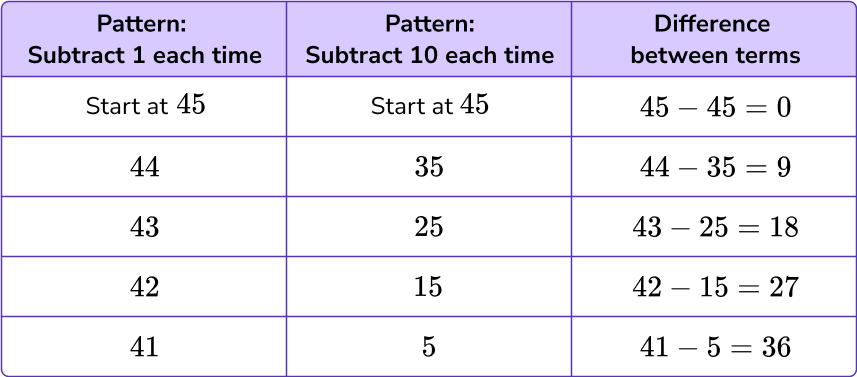The first difference is 0, because both patterns start at 45. Since one pattern -1 and one pattern -10, the -10 pattern will always subtract 9 more than the -1 pattern.

This is why the difference grows by 9 each time.

## Sequences FAQs

How do mathematical topics for sequences advance?

Students begin with basic sequences that use recursive rules (like the ones on this page). In later grades, students will learn to create polynomials that can be used to solve for the nth term (any term of the sequence).

This is done through learning to write explicit formulas and recursive formulas. After this, students learn to extend this skill to include arithmetic series and geometric series in high school.

Do the terms of a sequence always have to be natural numbers (positive integers)?

No, the standards start with these numbers, since students are most familiar with these operations. However, in middle school, sequences grow to include all types of rational numbers. This knowledge later grows to include sequences with complex numbers and work with summations.

Does a sequence have a certain number of terms?

Unless specified as a finite sequence, most sequences are considered to be infinite sequences, which means they go on forever. When working with sequences, depending on the context, you can use any given number of terms necessary to solve the problem at hand.

Do the terms of an arithmetic sequence have a particular order?

Yes, the ordered list of numbers that makes up a sequence will become a different sequence if the terms are altered in any way that changes the common ratio relationship between them.

• Angles
• Lines
• 2D shapes
• Triangles

## Still stuck?

At Third Space Learning, we specialize in helping teachers and school leaders to provide personalized math support for more of their students through high-quality, online one-on-one math tutoring delivered by subject experts.

Each week, our tutors support thousands of students who are at risk of not meeting their grade-level expectations, and help accelerate their progress and boost their confidence.